Adding and Subtracting Integers Visual Number Talk for deepening understanding of the behaviours of adding negative numbers.

## In This Set of Visual Number Talk Prompts…

Students will explore integer addition through a real world contextual situation involving moving a car up and down levels in a parking garage..

## Intentionality…

In today’s visual math talk, present each parking garage scenario one at a time. In each scenario, encourage students to model the equation using a number line and solve. The purpose of today’s math talk is to make some generalizations about the result of various addition and subtraction scenarios involving positive and negative integers.

## Visual Math Talk Prompt #1

Students will be prompted with:

This car is parked on level 5.

The car is moved down 6 levels.

What level is the car on now?

Write an equation to represent this scenario.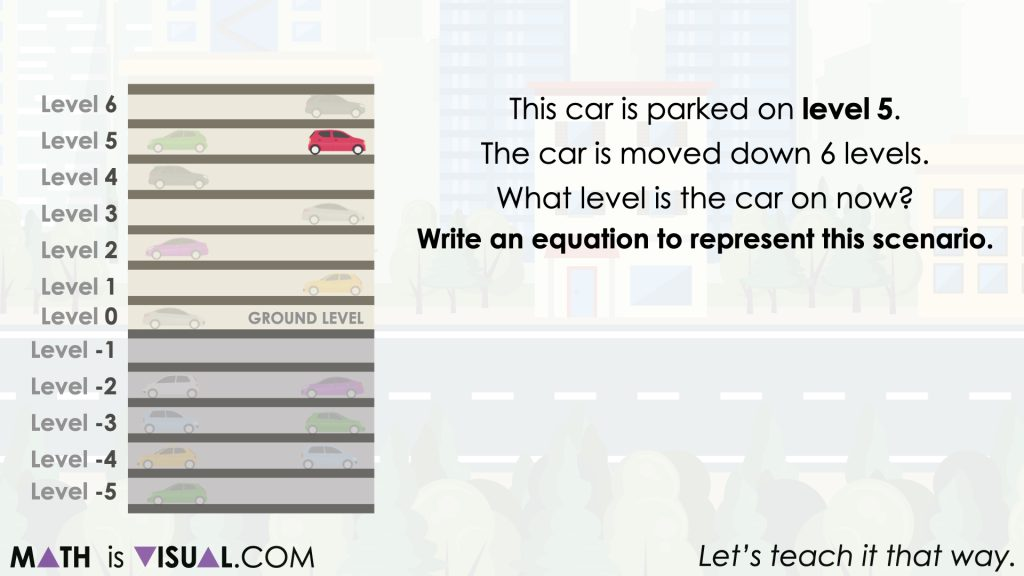In the first prompt from this string of related visual problems, students are asked to move the car down (or remove levels) from level 5 a total of 6 levels. The context was crafted so that it is clear that the car can move down below ground level and we’ll annotate those levels below ground using a negative sign.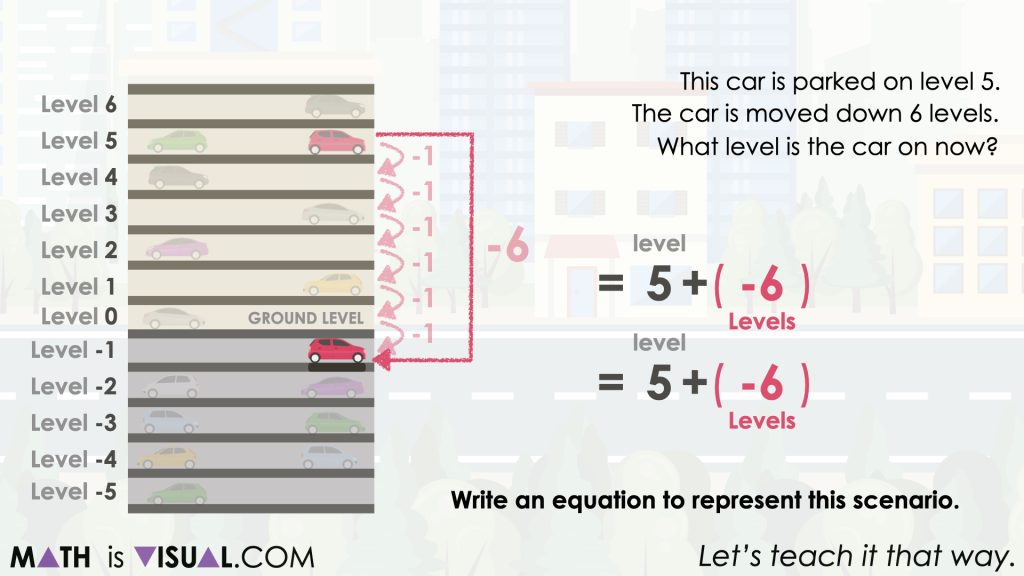When the car moves down 6 levels, we see there are only 5 levels until the ground floor (level 0) and one more level to go, bringing us to level -1.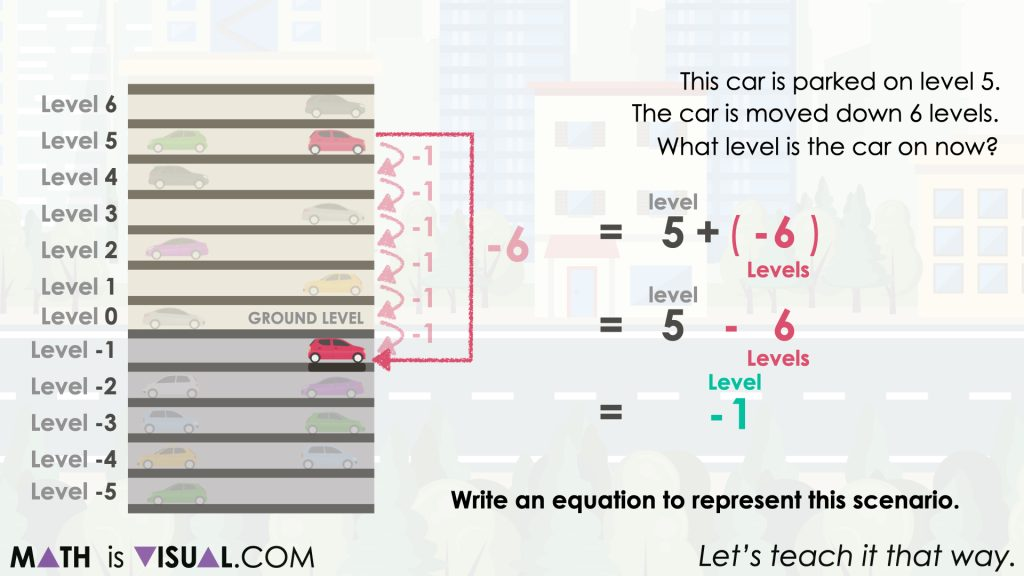Another approach is to leverage the zero principle by first decomposing -6 (or going down 6 levels) to -5 and -1 so level 5 and removing 5 levels cancel bringing the car to level 0. Then, we have a movement of -1 floors remaining to bring us to level -1.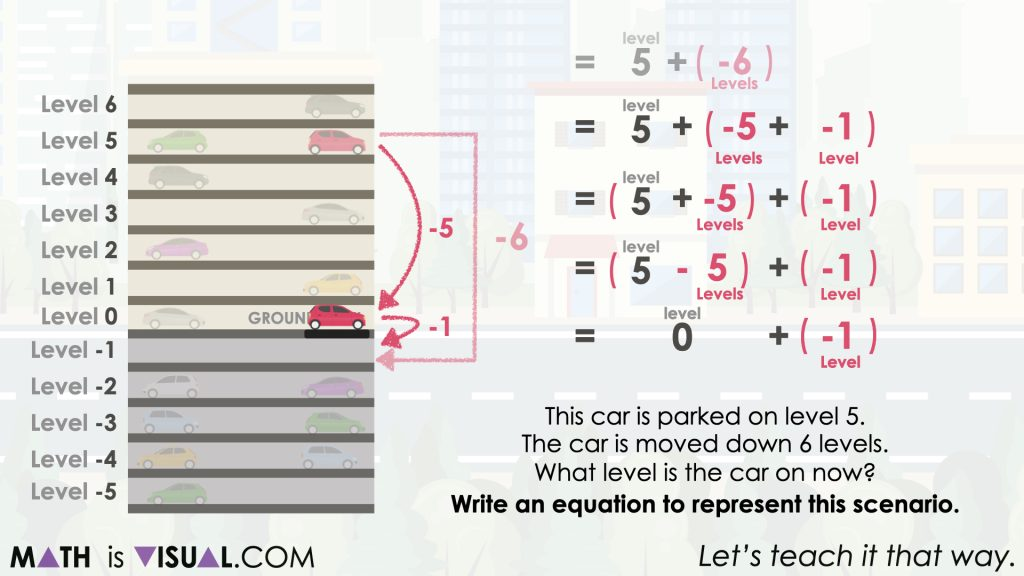Of course this action is very intuitive through the context of levels in a parking garage, however it is important to also annotate what we are doing using the symbolic representation for students to see the relationships involving integer operations of addition and subtraction.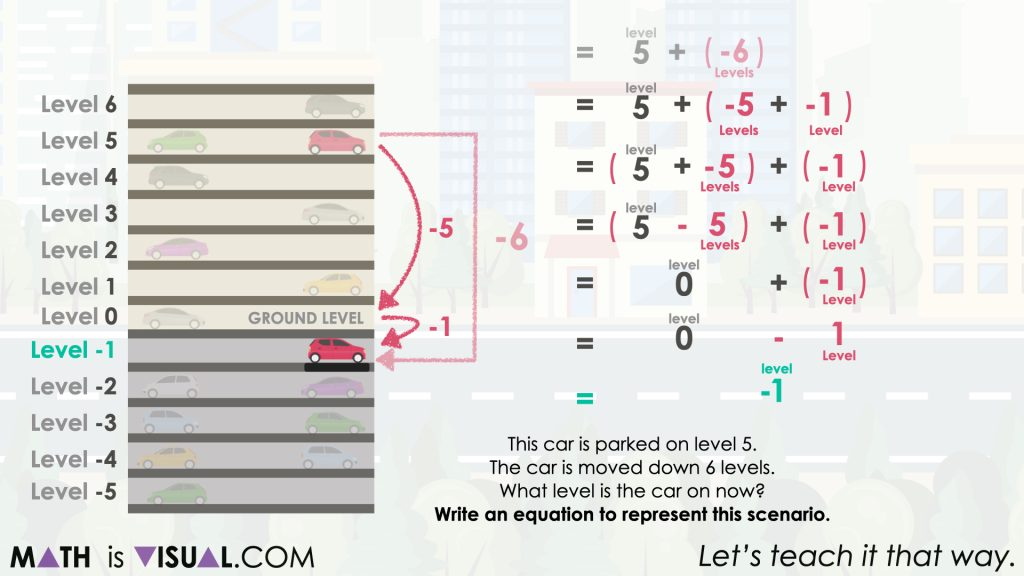Facilitator note:

The integers in this context can be interpreted as a change or as quantities of floors. For example, if the car starts on the fifth floor above ground level (5), and moves down 6 floors, this can be expressed as the addition of 6 negative floors [5 + (−6)], or a subtraction of 6 floors (5 − 6). Both statements result in the same answer (−1).

When a positive and a negative integer are added together, the result is negative if the absolute value of the positive integer is less than the absolute value of the negative integer.

## Want to Explore These Concepts & Skills Further?

Two (2) additional number talk prompts are available in Day 2 of the Going Up problem based math unit that you can dive into now.

Why not start from the beginning of this contextual 5-day unit of real world lessons from the Make Math Moments Problem Based Units page.

Did you use this in your classroom or at home? How’d it go? Post in the comments!

Math IS Visual. Let’s teach it that way.